# Connect Git through CCProxy

2017/09/09 19:48

1. Install Git on your computer.

2. Create User account for CCProxy (Figure 1).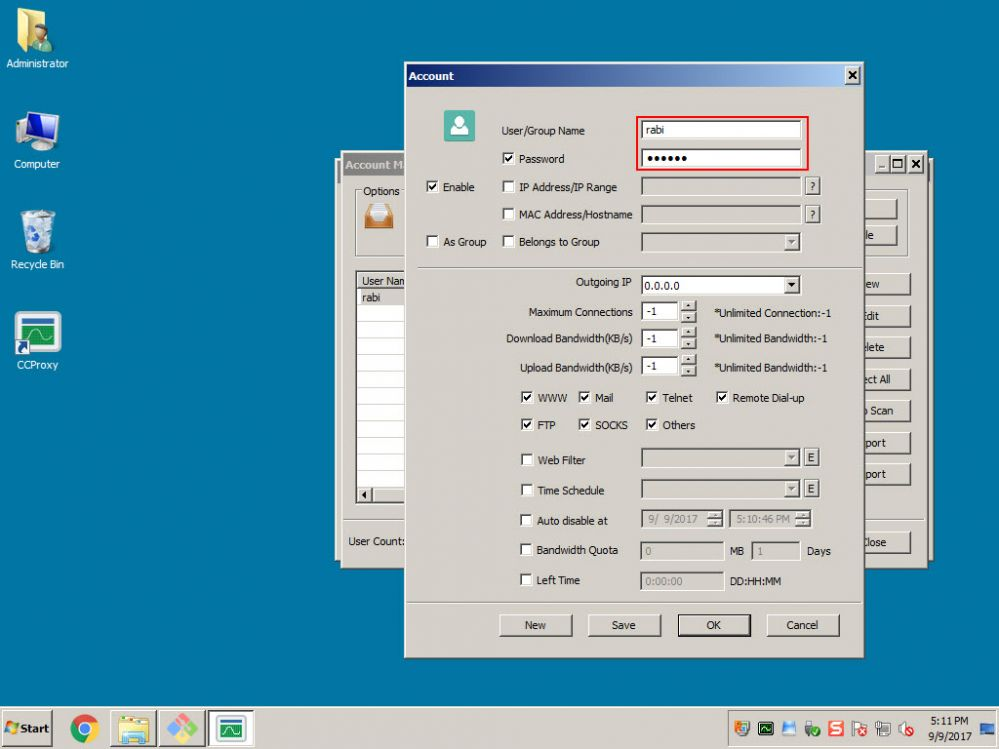Figure 1

3. Set the authentication to "User/Password" (Figure 2).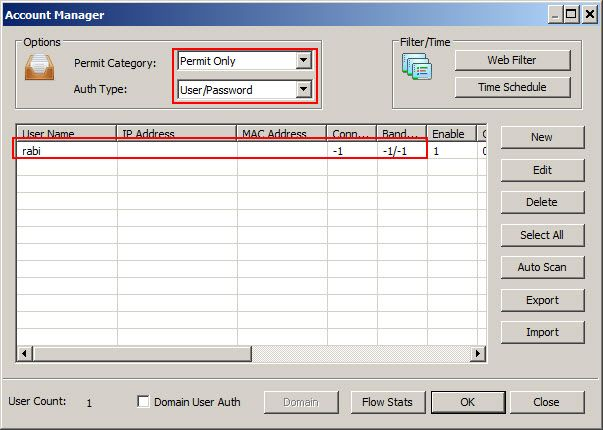Figure 2

4. Open Git bash and run the following command to add proxy settings for git (Figure 3).

Example:  git config --global http.proxy http://rabi:123456@192.168.1.251:808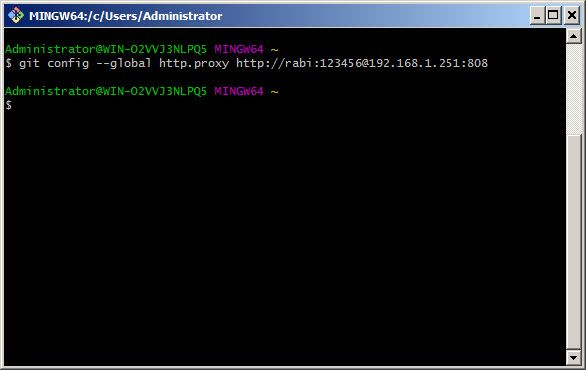Figure 3

5. To check if the proxy settings is set or not, run this command (Figure 4)

git config --get http.proxy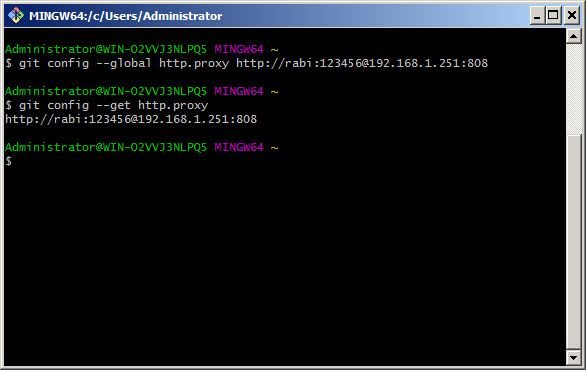Figure 4

6. Now, that proxy settings is set, lets initialize a local git repo which we can use to clone other repository (Figure 5)

git init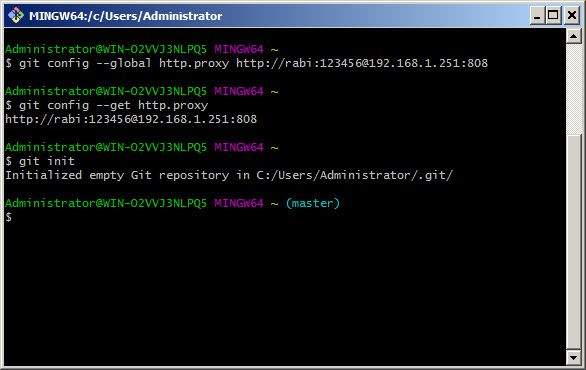Figure 5

7. Now, lets clone one of the git repo (Figure 6)Figure 6

8. Check the monitor window to make sure its going through CCProxy (Figure 7).Figure 7

Related: